Hydrol. Earth Syst. Sci., 26, 827–840, 2022
https://doi.org/10.5194/hess-26-827-2022
Hydrol. Earth Syst. Sci., 26, 827–840, 2022
https://doi.org/10.5194/hess-26-827-2022

Research article 15 Feb 2022

Research article | 15 Feb 2022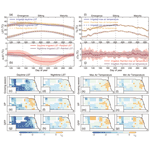# Untangling irrigation effects on maize water and heat stress alleviation using satellite data

Untangling irrigation effects on maize water and heat stress alleviation using satellite data
Peng Zhu and Jennifer Burney Peng Zhu and Jennifer Burney
• School of Global Policy and Strategy, University of California, San Diego, CA, USA

Correspondence: Peng Zhu (zhuyp678@gmail.com)

Abstract

Irrigation has important implications for sustaining global food production by enabling crop water demand to be met even under dry conditions. Added water also cools crop plants through transpiration; irrigation might thus play an important role in a warmer climate by simultaneously moderating water and high temperature stresses. Here we used satellite-derived evapotranspiration estimates, land surface temperature (LST) measurements, and crop phenological stage information from Nebraska maize to quantify how irrigation relieves both water and temperature stresses. Unlike air temperature metrics, satellite-derived LST revealed a significant irrigation-induced cooling effect, especially during the grain filling period (GFP) of crop growth. This cooling appeared to extend the maize growing season, especially for GFP, likely due to the stronger temperature sensitivity of phenological development during this stage. Our analysis also revealed that irrigation not only reduced water and temperature stress but also weakened the response of yield to these stresses. Specifically, temperature stress was significantly weakened for reproductive processes in irrigated maize. Attribution analysis further suggested that water and high temperature stress alleviation was responsible for 65±10 % and 35±5.3 % of the irrigation yield benefit, respectively. Our study underlines the relative importance of high temperature stress alleviation in yield improvement and the necessity of simulating crop surface temperature to better quantify heat stress effects in crop yield models. Finally, considering the potentially strong interaction between water and heat stress, future research on irrigation benefits should explore the interaction effects between heat and drought alleviation.

Share
1 Introduction

Irrigation – a large component of freshwater consumption sourced from water diversion from streams and groundwater (Wallace, 2000; Howell, 2001) – allows crops to grow in environments that do not receive sufficient rainfall, and buffers agricultural production from climate variability and extremes. Irrigated agriculture plays an outsized role in global crop production and food security: irrigated lands account for 17 % of total cropped area, yet they provide 40 % of global cereals (Rosegrant et al., 2002; Siebert and Döll, 2010). Meeting the rising food demands of a growing global population will require either increasing crop productivity and/or expansion of cropped areas; both strategies are daunting under projected climate change. Cropland expansion may be in marginal areas that require irrigation even in the present climate (Bruinsma, 2009); increasing temperatures will drive higher atmospheric vapor pressure deficits (VPDs) and raise crop water demand and crop water losses. This increasing water demand poses a water ceiling for crop growth and might necessitate irrigation application over present rainfed areas to increase or even maintain yields (DeLucia et al., 2019).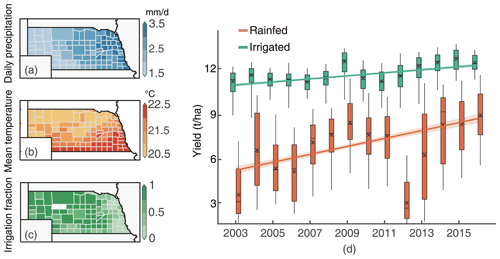Figure 1The spatial pattern of county-level multi-year (2003–2016) mean daily precipitation (a) and air temperature (b) during the maize growing season. County-level multi-year (2003–2016) mean maize irrigation fraction across Nebraska (c). The maize irrigation fraction is based on the USDA NASS report. Box plot of county-level irrigated and rainfed maize yield in Nebraska over the study period (d). The lines in (d) show the linear fitted yield trend with a 95 % confidence interval. Box plots indicate the median (horizontal line), mean (cross), interquartile range (box), and 5th–95th percentile (whiskers) of rainfed or irrigated yield across all counties.

However, the provision of additional irrigation water modifies both the land surface water and energy budgets. Additional water can result in an evaporative cooling effect, which may be beneficial for crop growth indirectly through lowering the frequency of extreme heat stress (Butler et al., 2018). High temperature stress will be more prevalent (Russo et al., 2014) under future warming and might result in more severe yield losses than water stress (Zhu et al., 2019) due to reduced photosynthesis, pollen sterility, and accelerated crop senescence in major cereals (Eyshi Rezaei et al., 2015; Rattalino Edreira et al., 2011; Ruiz-Vera et al., 2018). A better understanding of irrigation's potential to alleviate high temperature stress will therefore be important for agricultural management. More broadly, understanding how irrigation can or should contribute to a portfolio of agricultural adaptation strategies thus requires improved understanding of its relative roles in mitigating both water and heat stresses.

Climate models and meteorological data have been used to investigate how historical expansion of irrigation at global and regional scales has influenced the climate system, including surface cooling and precipitation variation (Kang and Eltahir, 2019; Thiery et al., 2017; Bonfils and Lobell, 2007; Sacks et al., 2009). However, many crop models still use air temperature rather than canopy temperature to estimate heat stress; this may overestimate heat stress effects in irrigated cropland (Siebert et al., 2017), since canopy temperature can deviate significantly from air temperature depending on the crop moisture conditions (Siebert et al., 2014). Recently, a comparison of crop-model-simulated canopy temperatures suggests that most crop models lack sufficient ability to reproduce the field-measured canopy temperature, even for models with a good performance in grain yield simulation (Webber et al., 2017).

Satellite-derived land surface temperature (LST) measurements have been used to directly quantify regional-scale surface warming or cooling effects resulting from surface energy budget changes due to changes in land cover and land management (Loarie et al., 2011; Tomlinson et al., 2012; Peng et al., 2014). Importantly, yield prediction model comparisons suggest that replacing air temperature with MODIS LST can improve yield predictions because LST accounts for both evaporative cooling and water stress (Li et al., 2019). Satellite data also provide the observational evidence to constrain model performance or directly retrieve crop growth status information. For example, satellite-derived soil moisture has been used to characterize irrigation patterns and improve irrigation quantity estimations (Felfelani et al., 2018; Lawston et al., 2017; Jalilvand et al., 2019; Zaussinger et al., 2019). Integration of satellite products like LST therefore have the potential to improve our understanding of how irrigation and climate change impact crop yields and thus provide guidance for farmers to optimize management decisions.

In this study, we focused on Nebraska, the third largest maize producer in the United States. Multi-year mean climate data showed that conditions have been drier in western areas and warmer in southern areas of the state (Fig. 1a and b). Importantly, Nebraska has historically produced a mixture of irrigated and rainfed maize that facilitated comparison (more than half, 56 %, of the Nebraska maize cropland was irrigated, with more irrigated maize in the western area – see Fig. 1c – according to the United States Department of Agriculture; USDA, 2018a). County yield data from the USDA showed that interannual fluctuations in rainfed maize yield have in general been much larger than for irrigated maize (Fig. 1b). Although irrigated yields were higher, rainfed maize yields grew faster than irrigated (an average of 3.9 % per year versus 1.0 % per year) over the study period (2003–2016) (Fig. 1b), in part because breeding technology progress has improved the drought tolerance of maize hybrids (Messina et al., 2011).

As noted above, irrigation potentially benefits crop yields by moderating both water and high temperature stress. Here we used satellite-derived LST and satellite-derived water stress metrics to statistically tease apart the contributions of irrigation to water and heat stress alleviation separately. We (1) evaluated the difference in temperature and moisture conditions over irrigated and rainfed maize croplands, (2) explored how irrigation mitigated water and high temperature stresses using panel statistical models, (3) quantified the relative contributions of irrigation-induced water and high temperature stress alleviation to yield improvements, and (4) explored whether current crop models reproduced the observed irrigation benefits for maize growth status.

2 Materials and methods

We first describe the data used, followed by a brief description of the statistical methodology.

## 2.1 Satellite products to identify irrigated and non-irrigated maize areas

We used the United States Department of Agriculture's Cropland Data Layer (CDL) to identify maize croplands for each year in the study period 2003–2016 (USDA, 2018b). The irrigation distribution map across Nebraska was obtained from a previous study that used Landsat-derived plant greenness and moisture information to create a continuous annual irrigation map across US Northern High Plains (Deines et al., 2017). The irrigation map showed very high accuracy (92 % to 100 %) when validated with randomly generated test points and was also highly correlated with county statistics (R2=0.88–0.96) (Deines et al., 2017). Both the CDL and irrigation map are at 30 m resolution. We first projected them to MODIS sinusoidal projection and then aggregated them to 1 km resolution to align with MODIS ET and LST products. Then, pixels containing more than 60 % maize and an irrigation fraction > 60 % were labeled as irrigated maize, while pixels with >60 % maize and <10 % irrigation fraction were labeled as rainfed maize croplands. As always, threshold selection involves a trade-off between mixing samples and retaining as many samples as possible. Our choices of <10 % as the threshold for rainfed maize and 60 % to define irrigated maize represented the best optimization in our sample, as we found that a more stringent threshold had a very small effect on LST differences between irrigated and rainfed maize at county level but resulted in significant data omission (more details in Figs. S1 and S2 in the Supplement).

## 2.2 Maize phenology information

Maize growth stage information derived in a previous study was used to assess the influence of irrigation on maize growth during different growth stages (Zhu et al., 2018). Stage information, including emergence date, silking date, and maturity date, was derived with MODIS WDRVI (Wide Dynamic Range Vegetation Index, 8 d and 250 m resolution) based on a hybrid method combining shape model fitting (SMF) and threshold-based analysis. Then we defined the vegetative period (VP) as the period from emergence date to silking date, the grain filling period (GFP) as the period from silking date to maturity date, and the growing season (GS) as the period from emergence date to maturity date. Details can be found in our previous studies (Zhu et al., 2018). WDRVI was used due to its higher sensitivity to changes at high biomass than other vegetation indices (Gitelson, 2004) and was estimated with the following equations:

$\begin{array}{}\text{(1)}& & \mathrm{NDVI}=\left({\mathit{\rho }}_{\mathrm{NIR}}-{\mathit{\rho }}_{\mathrm{red}}\right)/\left({\mathit{\rho }}_{\mathrm{NIR}}+{\mathit{\rho }}_{\mathrm{red}}\right),\text{(2)}& & \mathrm{WDRVI}=\mathrm{100}\cdot \frac{\left[\left(\mathit{\alpha }-\mathrm{1}\right)+\left(\mathit{\alpha }+\mathrm{1}\right)×\mathrm{NDVI}\right]}{\left[\left(\mathit{\alpha }+\mathrm{1}\right)+\left(\mathit{\alpha }-\mathrm{1}\right)×\mathrm{NDVI}\right]},\end{array}$

where ρred and ρNIR are the MODIS surface reflectance in the red and near-infrared (NIR) bands, respectively. To minimize the effects of aerosols, we used the 8 d composite products in MOD09Q1 and MYD09Q1 and quality-filtered the reflectance data using the band quality control flags. Only data passing the highest quality control were retained (Zhu et al., 2018). The scaling factor, α=0.1, was adopted based on a previous study to degrade the fraction of the NIR reflectance at moderate-to-high green vegetation and best linearly capture the maize green leaf area index (LAI) (Guindin-Garcia et al., 2012).

## 2.3 Temperature exposure during maize growth

We used daily 1 km spatial resolution MODIS Aqua LST (MYD11A1) data to characterize the crop surface temperature; since its overpassing times are at 01:30 and 13:30 LT, it is closer to the times of daily minimum and maximum temperature than the MODIS Terra LST (Wan, 2008) and is therefore better for characterizing crop surface temperature stress (Johnson, 2016; Li et al., 2019). For quality control, pixels with an LST error > 3 were filtered out based on the corresponding MODIS LST quality assurance layers. Missing values (less than 3 % of total observations) were interpolated with robust spline function (Teuling et al., 2010). Aqua LST data are available after July 2002; we thus restricted our study to the period 2003–2016. For comparison, we also obtained daily minimum and maximum surface air temperature (Tmin and Tmax) at 1 km resolution from Daymet version 3 (Thornton et al., 2018). For both MODIS LST and air temperature, we calculated integrated crop heat exposure – the growing degree days (GDD) and extreme degree days (EDD) – according to the following definitions.

$\begin{array}{}\text{(3)}& \begin{array}{rl}{\mathrm{GDD}}_{\mathrm{8}}^{\mathrm{30}}& =\sum _{t=\mathrm{1}}^{N}{\mathrm{DD}}_{t},\phantom{\rule{0.25em}{0ex}}{\mathrm{DD}}_{t}\\ & =\left\{\begin{array}{ll}\mathrm{0},& \mathrm{when}\phantom{\rule{0.25em}{0ex}}T<\mathrm{8}\phantom{\rule{0.125em}{0ex}}{}^{\circ }\mathrm{C}\\ T-\mathrm{8}& \mathrm{when}\phantom{\rule{0.25em}{0ex}}\mathrm{8}\phantom{\rule{0.125em}{0ex}}{}^{\circ }\mathrm{C}\le T<\mathrm{30}\phantom{\rule{0.125em}{0ex}}{}^{\circ }\mathrm{C}\\ \mathrm{22},& \mathrm{when}\phantom{\rule{0.25em}{0ex}}T\ge \mathrm{30}\phantom{\rule{0.125em}{0ex}}{}^{\circ }\mathrm{C}\end{array}\right\}\end{array}\end{array}$

$\begin{array}{}\text{(4)}& \begin{array}{rl}{\mathrm{EDD}}_{\mathrm{30}}^{\mathrm{\infty }}& =\sum _{t=\mathrm{1}}^{N}{\mathrm{DD}}_{t},\phantom{\rule{0.25em}{0ex}}{\mathrm{DD}}_{t}\\ & =\left\{\begin{array}{ll}\mathrm{0},& \mathrm{when}\phantom{\rule{0.25em}{0ex}}T<\mathrm{30}\phantom{\rule{0.125em}{0ex}}{}^{\circ }\mathrm{C}\\ T-\mathrm{30},& \mathrm{when}\phantom{\rule{0.25em}{0ex}}T\ge \mathrm{30}\phantom{\rule{0.125em}{0ex}}{}^{\circ }\mathrm{C}\end{array}\right\}\end{array}\end{array}$

Here, temperature (T) could be either air temperature or LST, interpolated from daily to hourly values with sine function (Tack et al., 2017). t represents the hourly time step, and N is the total number of hours in a specified growing period (either the entire growing season or a specific phenological growth phase, as defined below). Following previous studies (Lobell et al., 2011; Zhu et al., 2019), we used 30 C as the high temperature threshold, although higher values might be applicable in some settings (Sanchez et al., 2014).

## 2.4 Maize water stress

Water stress during maize growth was characterized by the ratio of evapotranspiration (ET) to potential evapotranspiration (PET), as in a previous study (Mu et al., 2013). We used MODIS products (MYD16A2) for both ET and PET based on its good performance for natural vegetation (Mu et al., 2011); however, our comparison using flux-tower-observed ET at an irrigated maize site in Nebraska suggested that ET for the irrigated maize was significantly underestimated by MODIS ET (Fig. S3). We therefore also used another ET product (SSEBop ET) to replace MODIS ET. SSEBop ET was also estimated with MODIS products (Senay et al., 2013), like LST, vegetation index, and albedo as input variables, but used a revised algorithm including predefined boundary conditions for hot and cold reference pixels (Senay et al., 2013) and showed better performance than MODIS ET (Velpuri et al., 2013). We also saw improved performance when we compared it with flux-tower-observed ET at an irrigated maize site (Fig. S4). The comparison of MODIS PET and flux-tower-estimated PET showed satisfactory performance for MODIS PET (Fig. S5). Since MODIS PET from MYD16A2 has a spatial resolution of 500 m with 8 d temporal resolution, while SSEBop ET has 1 km spatial resolution with daily time step, we reconciled the two datasets to 1 km spatial resolution and 8 d temporal resolution.

## 2.5 Crop model simulation results

We compared the results of our statistical analysis with four gridded crop models. Simulation results from pAPSIM, pDSSAT, LPJ-GUESS, and CLM-crop for both rainfed and irrigated maize across Nebraska were obtained from the Agricultural Model Intercomparison and Improvement Project (AgMIP) (Rosenzweig et al., 2013) and Inter-Sectoral Impact Model Intercomparison Project 1 (ISIMIP1) (Warszawski et al., 2014). The four models were driven by the same climate forcing dataset (AgMERRA) and run at a spatial resolution of 0.5 arcdegree longitude and latitude. All simulations were conducted for purely rainfed and nearly perfectly irrigated conditions. These models simulated maize yield, total biomass, ET, and growing stage information (planting date, flowering date, and maturity date). Planting date occurs on the first day following the prescribed sowing date on which soil temperature is at least 2 above the 8 C base temperature. Harvest occurs once the specified heat units are reached. Heat units to maturity were calibrated from the prescribed crop calendar data (Elliott et al., 2015). Crop model simulation was evaluated by calculating the Pearson correlation between simulated yields in the baseline simulations and detrended historical yields for each county from the Food and Agriculture Organization. The management scenario “harmnon” was selected, meaning the simulation using harmonized fertilizer inputs and assumptions on growing seasons. More details on the simulation protocol can be found in Elliott et al. (2015) and Müller et al. (2019). We used this model comparison project outputs to shed light on how well crop models simulated the irrigation benefits we identified in different phases of crop growth.

## 2.6 Method

We used standard panel statistical analysis techniques to identify the impacts of irrigation on maize productivity via heat stress reduction and water stress reduction pathways.

Comparison of LST, ET, PET, ET $/$ PET, GDD, and EDD between irrigated and rainfed maize areas was performed within each county to minimize the effects of other spatially varying factors, like background temperature and management practices, on surface temperature and evapotranspiration. These biophysical variables (LST, ET, PET, ET $/$ PET, GDD, and EDD) averaged over each county were then integrated over the vegetative period (VP, from emergence date to silking date), grain filling period (GFP, from silking date to maturity date), and whole growing season (GS, from emergence date to maturity date) so we could evaluate whether and how irrigation had differentially influenced maize growth during early VP and late GFP.

We further examined how irrigation had changed the sensitivity of maize yield and its components to temperature variation. As done in our previous study (Zhu et al., 2019), we decomposed the total yield (t ha−1) variation into three components: biomass growth rate (BGR, t ha−1 d−1), growing season length (GSL, days), and harvest index (HI) based on the following equation:

$\begin{array}{}\text{(5)}& \mathrm{Yield}=\mathrm{HI}\cdot \mathrm{AGB}=\mathrm{HI}\cdot \mathrm{BGR}\cdot \mathrm{GSL}.\end{array}$

Aboveground biomass (AGB, t ha−1) was retrieved through a regression model:

$\begin{array}{}\text{(6)}& \mathrm{AGB}=\mathit{\beta }\cdot {\mathrm{IWDRVI}}^{\mathit{\gamma }},\end{array}$

which was built in the previous study by regressing field-measured maize AGB against MODIS-derived integrated WDRVI (IWDRVI) (Zhu et al., 2019). The coefficients β and γ were estimated as 16.4±2.5 (t ha−1) and 0.8±0.08. Then HI could be estimated as yield $/$ AGB and BGR could be estimated as AGB $/$ GSL. This decomposition allowed us to examine how different crop growth physiological processes responded to external forcing: HI characterizes dry matter partitioning between source organ and sink organ and is mainly related to processes determining grain size and grain weight; BGR is related to physiological processes of daily carbon assimilation rate through photosynthesis, and GSL is related to crop phenological development. The uncertainties in AGB estimation result from the parameters in the regression model (Eq. 6) converting IWDRVI to AGB. Here we quantified the uncertainties rooted in the estimated parameters by running the panel model 1000 times with the samples generated from each parameter's 95 % confidence interval (Zhu et al., 2019).

Temperature sensitivity of irrigated or rainfed yield (${S}_{T}^{\mathrm{Yield}}$) was estimated using a panel data model (Eq. 7) with growing season mean LST and ET $/$ PET as the explanatory variables:

$\begin{array}{}\text{(7)}& \mathrm{log}\left({\mathrm{Yield}}_{i,t}\right)={\mathit{\gamma }}_{\mathrm{1}}t+{\mathit{\gamma }}_{\mathrm{2}}{\mathrm{LST}}_{i,t}+{\mathit{\gamma }}_{\mathrm{3}}{\frac{\mathrm{ET}}{\mathrm{PET}}}_{i,t}+{\mathrm{County}}_{i}+{\mathit{\epsilon }}_{i,t}.\end{array}$

Yieldi,t is maize yield (t ha−1) in county i and year t. It is a function of overall yield trends (γ1t) that fairly steadily increased over the study period (Fig. 1b), local crop temperature stress (LSTi,t), and local crop water stress (${\frac{ET}{PET}}_{i,t}\right)$. The Countyi terms provide an independent intercept for each county (fixed effect) and thus account for time-invariant county-level differences that contributed to variations in yield, like the soil quality. εi,t is an idiosyncratic error term. γ2 or $\frac{\partial \mathrm{ln}\left(\mathrm{Yield}\right)}{\partial \mathrm{LST}}$ defines the temperature sensitivity of yield. The temperature sensitivity of BGR (${S}_{T}^{\mathrm{BGR}}$), HI (${S}_{T}^{\mathrm{HI}}$), and GSL (${S}_{T}^{\mathrm{GSL}}$) could be estimated with Eq. (7) in a similar way by using BGR, HI, and GSL as dependent variables. Here, the dependent variable Yield (BGR, GSL, and HI) was logged, so the estimated temperature sensitivity represented the percentage change in Yield (BGR, GSL, and HI) with a 1 C temperature increase.

To quantify the relative contribution of water and high temperature stress alleviation to yield benefit, we related the yield difference between irrigated and non-irrigated maize (irrigation yield-rainfed yield, ΔYield) to a quadratic function of growing season EDD and ET $/$ PET differences between irrigated and rainfed maize:

$\begin{array}{}\text{(8)}& \begin{array}{rl}\mathrm{\Delta }{\mathrm{Yield}}_{i,t}& ={\mathit{\gamma }}_{\mathrm{1}}\mathrm{\Delta }{\frac{\mathrm{ET}}{\mathrm{PET}}}_{i,t}+{\mathit{\gamma }}_{\mathrm{2}}\mathrm{\Delta }{\frac{\mathrm{ET}}{\mathrm{PET}}}_{i,t}^{\mathrm{2}}+{\mathit{\gamma }}_{\mathrm{3}}\mathrm{\Delta }{\mathrm{EDD}}_{i,t}\\ & +{\mathit{\gamma }}_{\mathrm{4}}\mathrm{\Delta }{\mathrm{EDD}}_{i,t}^{\mathrm{2}}+{\mathrm{County}}_{i}+{\mathit{\epsilon }}_{i,t}.\end{array}\end{array}$

The yield improvement explained by heat and water stress alleviation was estimated as

$\frac{{\mathit{\gamma }}_{\mathrm{1}}\sum \mathrm{\Delta }{\frac{\mathrm{ET}}{\mathrm{PET}}}_{i,t}+{\mathit{\gamma }}_{\mathrm{2}}\sum \mathrm{\Delta }{\frac{\mathrm{ET}}{\mathrm{PET}}}_{i,t}^{\mathrm{2}}+{\mathit{\gamma }}_{\mathrm{3}}\sum \mathrm{\Delta }{\mathrm{EDD}}_{i,t}+{\mathit{\gamma }}_{\mathrm{4}}\sum \mathrm{\Delta }{\mathrm{EDD}}_{i,t}^{\mathrm{2}}}{\sum \mathrm{\Delta }{\mathrm{Yield}}_{i,t}}.$

The relative contribution of water and high temperature stress alleviation was estimated as

$\frac{{\mathit{\gamma }}_{\mathrm{1}}\sum \mathrm{\Delta }{\frac{\mathrm{ET}}{\mathrm{PET}}}_{i,t}+{\mathit{\gamma }}_{\mathrm{2}}\sum \mathrm{\Delta }{\frac{\mathrm{ET}}{\mathrm{PET}}}_{i,t}^{\mathrm{2}}}{{\mathit{\gamma }}_{\mathrm{1}}\sum \mathrm{\Delta }{\frac{\mathrm{ET}}{\mathrm{PET}}}_{i,t}+{\mathit{\gamma }}_{\mathrm{2}}\sum \mathrm{\Delta }{\frac{\mathrm{ET}}{\mathrm{PET}}}_{i,t}^{\mathrm{2}}+{\mathit{\gamma }}_{\mathrm{3}}\sum \mathrm{\Delta }{\mathrm{EDD}}_{i,t}+{\mathit{\gamma }}_{\mathrm{4}}\sum \mathrm{\Delta }{\mathrm{EDD}}_{i,t}^{\mathrm{2}}}$

and

$\frac{{\mathit{\gamma }}_{\mathrm{3}}\sum \mathrm{\Delta }{\mathrm{EDD}}_{i,t}+{\mathit{\gamma }}_{\mathrm{4}}\sum \mathrm{\Delta }{\mathrm{EDD}}_{i,t}^{\mathrm{2}}}{{\mathit{\gamma }}_{\mathrm{1}}\sum \mathrm{\Delta }{\frac{\mathrm{ET}}{\mathrm{PET}}}_{i,t}+{\mathit{\gamma }}_{\mathrm{2}}\sum \mathrm{\Delta }{\frac{\mathrm{ET}}{\mathrm{PET}}}_{i,t}^{\mathrm{2}}+{\mathit{\gamma }}_{\mathrm{3}}\sum \mathrm{\Delta }{\mathrm{EDD}}_{i,t}+{\mathit{\gamma }}_{\mathrm{4}}\sum \mathrm{\Delta }{\mathrm{EDD}}_{i,t}^{\mathrm{2}}},$

respectively. We also ran the model above using daytime LST difference (ΔLST) in lieu of ΔEDD as a robustness check:

$\begin{array}{}\text{(9)}& \begin{array}{rl}\mathrm{\Delta }{\mathrm{Yield}}_{i,t}& ={\mathit{\gamma }}_{\mathrm{1}}\mathrm{\Delta }{\frac{\mathrm{ET}}{\mathrm{PET}}}_{i,t}+{\mathit{\gamma }}_{\mathrm{2}}\mathrm{\Delta }{\frac{\mathrm{ET}}{\mathrm{PET}}}_{i,t}^{\mathrm{2}}+{\mathit{\gamma }}_{\mathrm{3}}\mathrm{\Delta }{\mathrm{LST}}_{i,t}\\ & +{\mathit{\gamma }}_{\mathrm{4}}\mathrm{\Delta }{\mathrm{LST}}_{i,t}^{\mathrm{2}}+{\mathrm{County}}_{i}+{\mathit{\epsilon }}_{i,t}.\end{array}\end{array}$

To diagnose any potential collinearity between $\mathrm{\Delta }\frac{\mathrm{ET}}{\mathrm{PET}}$ and ΔLST, we calculated the variance inflation factor (VIF) for the model above. In this formulation the relative contributions of water and high temperature stress alleviation were estimated as

$\frac{{\mathit{\gamma }}_{\mathrm{1}}\sum \mathrm{\Delta }{\frac{\mathrm{ET}}{\mathrm{PET}}}_{i,t}+{\mathit{\gamma }}_{\mathrm{2}}\sum \mathrm{\Delta }{\frac{\mathrm{ET}}{\mathrm{PET}}}_{i,t}^{\mathrm{2}}}{{\mathit{\gamma }}_{\mathrm{1}}\sum \mathrm{\Delta }{\frac{\mathrm{ET}}{\mathrm{PET}}}_{i,t}+{\mathit{\gamma }}_{\mathrm{2}}\sum \mathrm{\Delta }{\frac{\mathrm{ET}}{\mathrm{PET}}}_{i,t}^{\mathrm{2}}+{\mathit{\gamma }}_{\mathrm{3}}\sum \mathrm{\Delta }{\mathrm{LST}}_{i,t}+{\mathit{\gamma }}_{\mathrm{4}}\sum \mathrm{\Delta }{\mathrm{LST}}_{i,t}^{\mathrm{2}}}$

and

$\frac{{\mathit{\gamma }}_{\mathrm{3}}\sum \mathrm{\Delta }{\mathrm{LST}}_{i,t}+{\mathit{\gamma }}_{\mathrm{4}}\sum \mathrm{\Delta }{\mathrm{LST}}_{i,t}^{\mathrm{2}}}{{\mathit{\gamma }}_{\mathrm{1}}\sum \mathrm{\Delta }{\frac{\mathrm{ET}}{\mathrm{PET}}}_{i,t}+{\mathit{\gamma }}_{\mathrm{2}}\sum \mathrm{\Delta }{\frac{\mathrm{ET}}{\mathrm{PET}}}_{i,t}^{\mathrm{2}}+{\mathit{\gamma }}_{\mathrm{3}}\sum \mathrm{\Delta }{\mathrm{LST}}_{i,t}+{\mathit{\gamma }}_{\mathrm{4}}\sum \mathrm{\Delta }{\mathrm{LST}}_{i,t}^{\mathrm{2}}},$

respectively.

3 Results

As expected, irrigation improved maize yield and the yield benefit showed a distinct spatial variation when we compared areas we identified as irrigated versus rainfed maize. The yield benefit of irrigation was much higher in the western area of the state (Fig. 2a) because the drier environment in the western area featured a wider yield gap between irrigated and rainfed cropland in an average year. The satellite-derived vegetation index WDRVI reflected these differences, with higher values in areas we identified as irrigated maize, especially around maize silking (Fig. 2b). Importantly, this suggested that irrigated and rainfed croplands were distinguishable based on satellite-derived crop seasonality information.Figure 2The difference between irrigated and rainfed maize yield (a) and satellite-observed vegetation index (b, c). The shaded area in (b) and (c) shows 1 standard deviation of the WDRVI (b) and WDRVI difference (c).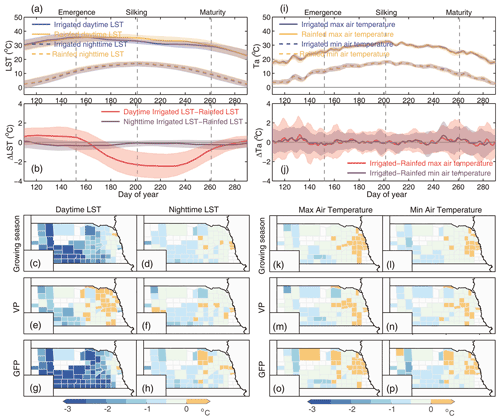Figure 3Spatial–temporal patterns of daytime and nighttime MODIS LST differences (a–h) and surface air temperature differences (i–p) between irrigated and rainfed maize in different growth stages: vegetative period and grain filling period. The shaded areas in (a), (b), (i), and (j) show 1 standard deviation of corresponding variables.

When county-level LST data were averaged over 2003–2016, the daytime LST in irrigated maize was 1.5 cooler than rainfed maize, while nighttime LST showed a very slight difference (0.2) (Fig. 3a and b). When the LST differences were integrated over different growing periods (Fig. 3e–h), we found that the daytime cooling effect was greatest in the GFP (Fig. 3g), probably due to the higher LAI (or ground cover) and transpiration during that stage of growth. This was also consistent with previous field studies showing that irrigation was mainly applied during the middle to late reproductive period, which corresponded to the greatest water demand period (Chen et al., 2018). The spatial pattern of the LST difference showed a stronger cooling effect in the western area (Fig. 3c–h), which was similar to the spatial pattern of yield benefit identified in Fig. 2a. In contrast, surface air temperature showed a much smaller daytime cooling effect (Fig. 3i and j). The mean daytime and nighttime air temperature differences between irrigated and rainfed maize were −0.2 and −0.3, respectively, and the spatial pattern of air temperature difference over VP and GFP was also relatively small between counties and crop growth periods (Fig. 3k–p). The difference between spatial–temporal patterns identified using LST and air temperature likely arises because LST reflects canopy energy partition between the latent heat flux and sensible heat flux. Additional moisture provided by irrigation results in more heat transferred as latent heat flux, creating a cooling effect.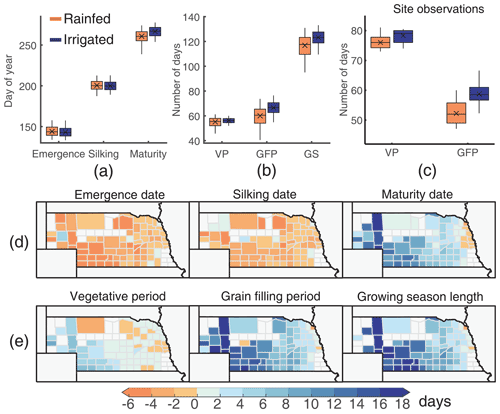Figure 4Box plot of maize phenological date (a) and duration (b, c) for irrigated and rainfed maize areas. The spatial pattern of phenological date and duration differences between irrigated and rainfed maize areas (d, e).

Temperature is an important driver of crop phenology and has been used as the primary environmental variable in crop phenology models (Wang and Engel, 1998). Given the identified irrigation cooling, we further examined how irrigation altered maize phenological stages. We found that irrigated maize showed an earlier emergence and silking but delayed maturity (Fig. 4a). Consequently, GFP was extended by 7.5 d on average, which contributed to most of the total GS extension (8.1 d) (Fig. 4b). Site measurements of phenological stage information confirmed that irrigated maize had a longer GS, especially during GFP (Fig. 4c). That this extension mainly occurred during GFP could be due to the fact that (1) LST cooling was more prominent during GFP, (2) phenological development during GFP was more sensitive to temperature variation than development during VP (Egli, 2004), and there were (3) variety differences between irrigated and rainfed maize. The spatial pattern suggested that GS and GFP extension was more significant in the western area of the state (Fig. 4g and h), likely due to the corresponding stronger cooling effect.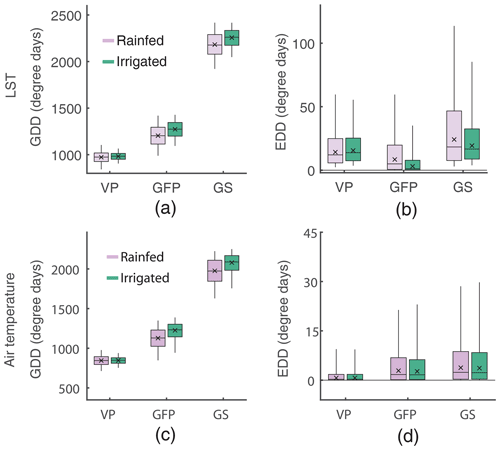Figure 5Box plot of GDD and EDD estimated with MODIS LST (a, b) and surface air temperature (c, d) for irrigated and rainfed maize areas. Box plots indicate the mean (cross), median (horizontal line), 25th–75th percentile (box), and 5th–95th percentile (whiskers) of corresponding variables in all year and county combinations.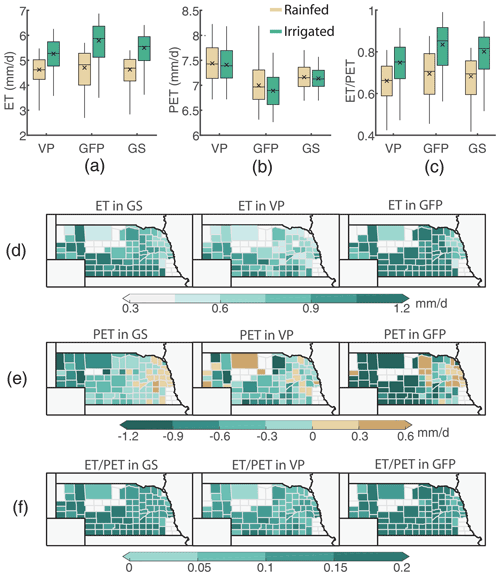Figure 6Box plot of SSEBop ET, MODIS PET, and ET $/$ PET for irrigated and rainfed maize areas (a–c). Spatial pattern of SSEBop ET, MODIS PET, and ET $/$ PET differences between irrigated and rainfed maize areas (d–f).

We integrated LST or air temperature as described above (Sect. 2.3) to estimate total heat exposure (GDD and EDD) over the maize growing season. We found that both LST and air-temperature-estimated GDD were greater in irrigated maize than GDD in rainfed maize across most counties, especially during GFP (Fig. 5a and c), which was very likely due to the GFP extension. As GDD characterizes the beneficial thermal time accumulation, the greater GDD in irrigated maize might contribute to the higher yield. In terms of EDD, LST-estimated EDD suggested that irrigation suppressed high temperature stress, especially for GFP (Fig. 5b), while air-temperature-estimated EDD failed to characterize the irrigation-induced lower high temperature stress (Fig. 5d).

SSEBop ET and MODIS PET were used to explore how irrigation influenced water demand and water supply across maize. We found that irrigation led to 27 % higher (p<0.001) ET and 2 % lower (p>0.05) PET (Fig. 6a and b). Higher ET was anticipated in irrigated maize, and lower PET might be due to the irrigation cooling effect, which resulted in lower VPD and thus lower evaporative demand. We used the ratio of ET to PET as a proxy for water stress in this study, whereby low values indicated that plants were not transpiring at their full potential in the ambient conditions. This ratio was higher for irrigated maize, especially during the GFP (Fig. 6c), and the spatial distribution suggested that the difference was greater in western counties than eastern counties (Fig. 6d and e), similar to the distribution of the local cooling effect identified in Fig. 3c.

We divided the temperature sensitivity of yield into three components (sensitivity of BGR, GSL, and HI) to investigate how irrigation changed the response of maize physiological processes to temperature. Because collinearity between LST and ET $/$ PET was potentially worrisome, we quantified the variance inflation factor (VIF) in the model; this was found to be well below standard thresholds, with a value of 2.8 and 3.6 for irrigated and rainfed maize yield, respectively. (VIFs over 10 indicate strongly collinear variables, with 5 being a more strict standard.) As shown in Fig. 7, we found that temperature sensitivity of yield was significantly weakened from −6.9 % per degree (p<0.01) to −1 % per degree (p<0.01) in rainfed vs. irrigated areas, and this yield sensitivity change was mainly driven by a change in the sensitivity of the HI, which was weakened from −4.2 % per degree (p<0.01) to 1 % per degree (p<0.01). In both rainfed and irrigated maize, temperature sensitivity of GSL was quite close at approximately −2 % per degree (p<0.01), while BGR was only slightly influenced by temperature (Fig. 7).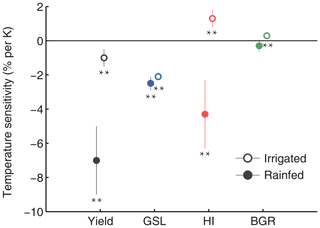Figure 7Temperature sensitivity of yield and yield components (GSL, HI, and BGR) for irrigated and rainfed maize areas. The error bars represent the 95 % confidence interval of estimated temperature sensitivity. Double asterisks (${}^{**}$) indicate a significant estimation of temperature sensitivity with p<0.01, while a single asterisk (*) indicates significance with p<0.05.

We found that irrigation not only lowered water and high temperature stress, but also made yield less sensitive to water and high temperature stress (Fig. 8a–c), consistent with previous studies (Troy et al., 2015; Tack et al., 2017). For example, field data across Africa suggest that better water management can reduce yield loss due to heat stress from −1.7 % per degree day to −1 % per degree day (Lobell et al., 2011). We statistically related yield differences to climatic variables differences using the linear model (Eq. 8) and estimated that 61±9.4 % of yield improvement between irrigated and rainfed maize could be explained by the irrigation-induced heat and water stress alleviation. We further calculated that 79±13 % of that yield improvement was due to water stress alleviation and 21±3.2 % was due to heat stress alleviation. Because the distribution of ΔEDD was truncated for points with ΔEDD>0 (Fig. 8e), we explored an alternative model with quadratic functions of ΔLST and $\mathrm{\Delta }\mathrm{ET}/\mathrm{PET}$ (Eq. 9). In this specification, 72±12 % of yield improvement was explained by water and high temperature stress alleviation, with 65±10 % and 35±5.3 % of yield improvement due to water and high temperature stress alleviation, respectively. We also estimated VIF in the model; this was found to be well below standard thresholds, with a value of 2.2. Intuitively, our low VIF value was likely due to the use of differences in LST and ET $/$ PET between irrigated and rainfed maize rather than directly using LST and ET $/$ PET as the explanatory variables. We also note that the high temperature stress alleviation estimated here appears larger than the estimation in a recent study (Li et al., 2019) wherein LST was also employed to detect the yield benefit of the irrigation cooling effect. But this is due to the fact that we estimated cooling effect benefits relative to the total sum of cooling and water stress effects, whereas Li et al. (2019) calculated the cooling effect relative to net yield differences between irrigated and rainfed maize. Since other effects (like cultivar difference and fertilizer application) might also contribute to the yield difference between irrigated and rainfed maize, the denominator used in Li et al. (2019) was larger.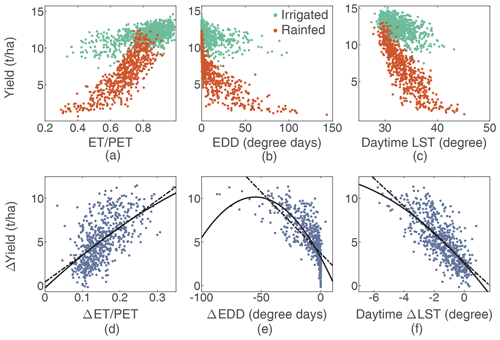Figure 8Response of maize yield to ET $/$ PET (a), EDD (b), and daytime LST (c) in both irrigated and rainfed maize. Response of yield differences to ET/PET (d), EDD (e), and daytime LST (f) differences between irrigated and rainfed maize. The linear (dash black line) and quadratic (solid black line) response curves of ΔYield to $\mathrm{\Delta }\mathrm{ET}/\mathrm{PET}$, ΔEDD, and ΔLST are shown in (d)(f).

Because we found a strong effect on yields via alleviation of heat stress (and not simply water stress), we compared our results with four process-based crop models that simulated crop growth under both rainfed and irrigated conditions. These simulations qualitatively reproduced the irrigation-induced higher maize yield, biomass, and ET (Fig. 9) but to different degrees. The highest modeled improvement was identified in CLM-crop, with increases of 57 %, 43 % and 32 % in yield, biomass and ET, respectively. However, all models except CLM-crop failed to reproduce the growing stage extension under irrigation (Fig. 9), likely because CLM-crop was the only one of the tested models to have implemented a canopy energy balance module to simulate canopy temperature. CLM-crop was thus the only model able to capture the irrigation-induced evaporative cooling effect (heat stress reduction). That the best agreement between observed and modeled results occurred with the only model that plausibly accounted for heat stress alleviation due to irrigation was further evidence that this was the phenomenon we captured in our satellite observational study.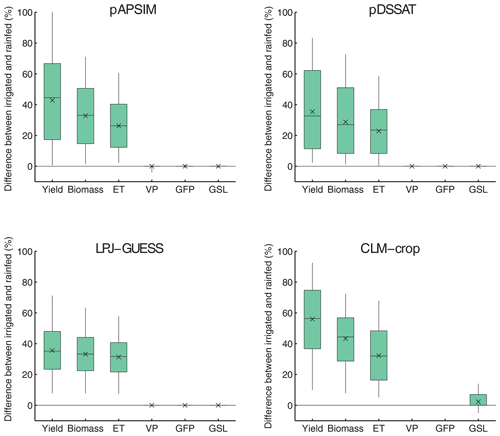Figure 9Box plot of crop-model-simulated yield, biomass, ET, and phenological duration (VP, GFP, and GSL) differences between irrigated and rainfed maize areas. For phenological duration, CLM-crop only reports GSL.

4 Discussion and conclusion

By integrating satellite products and ground-based information on cropping and irrigation, we showed that irrigated maize yields were higher than rainfed maize yields because added irrigation water reduced heat stress in addition to water stress. Our study underlines the relative importance of heat stress alleviation in yield improvement and the necessity of incorporating crop canopy temperature models to better characterize heat stress impacts on crop yields (Teixeira et al., 2013; Kar and Kumar, 2007). In addition, disentangling the two effects allows crop models to better predict crop phenology, considering that the irrigation-induced cooling effect alters maize growing phases.

Although ours is not the first study to suggest replacing air temperature with MODIS LST for maize yield prediction, especially under extreme warm and dry conditions, our results underscore important implications of doing so. Given the important role of heat stress in determining crop yield, thermal-band-derived LST information at finer spatial and temporal resolution should be a critical input for satellite-data-driven yield prediction models (Wang et al., 2015; Huryna et al., 2019; Li et al., 2019; Meerdink et al., 2019). In addition, given the differential responses of crop growth to heat and water stresses in different stages, fusing satellite-derived crop stage information with the heat and water stressors might improve crop yield prediction.

This study also has useful implications for process-based crop model development. In our model evaluation, only the model that implemented a canopy energy balance scheme captured the observed maize growth stage extension. Our results suggest that the heat stress alleviation due to irrigation identified here is largely overlooked in current crop models. As such, when those crop models are calibrated to match observed yields, processes associated with water stress alleviation are probably overestimated, resulting in uncertainties for predicting future irrigation water demand and crop yield. These uncertainties might mislead future adaptation decisions due to incomplete or biased estimates of the relative contributions of heat and water stress.

Relatedly, recent studies have compared heat stress representation in crops models which explicitly simulate canopy temperature (Webber et al., 2017). For example, STICS estimates canopy temperature using canopy energy balances which account for net radiation, soil heat flux, evapotranspiration, and aerodynamic resistance (Brisson et al., 2003). In APSIM, canopy temperature is taken as 6 C higher than air temperature when the crop is fully stressed and 6 C cooler than air temperature when the crop is fully transpiring. Between these limits, the basis of the expression for canopy temperature is the relationship between temperature difference (canopy temperature minus air temperature) and the ratio of actual and potential evapotranspiration (Webber et al., 2017). This model comparison study suggests that models using canopy temperature to account for heat stress effects indeed outperform models depending on air temperature, but the model comparison also identified a wide range for the simulated canopy temperature in current crop models. Therefore, assimilating satellite-derived LST might be a potential solution to improving crop models' heat stress representation so that they can better reproduce the observed heat stress effects (Meng et al., 2009; Xu et al., 2011). These remotely sensed LSTs can also be used to validate model-simulated LST, especially given that the recent ECOsystem Spaceborne Thermal Radiometer Experiment on Space Station (ECOSTRESS) mission makes hourly plant temperature measurements available (Meerdink et al., 2019). However, it is worth noting that the availability of satellite LST presents a constraint when thinking about future climate change impact studies. In addition, some caution is required for validating model-simulated LST, since LST is sensor- and satellite-specific.

Several limitations and caveats apply to our study. First, the daily MODIS daytime LST we used to explain crop maximum daily temperature had missing values due to quality control checks and was derived from a mix of crop cover and other land surface temperature information, which might bias the identified irrigation cooling effect. Specifically, using MODIS daytime LST as a proxy for true (measured) maximum crop surface temperature in an empirical statistical model might underestimate the benefit of the cooling effect (measurement error in a predictor variable producing attenuation bias). These uncertainties in the LST dataset might be resolved with the recently launched ECOSTRESS mission, as its hourly revisiting frequency enables better estimation of maximum daily temperature. The second issue is that water stress and heat stress are not perfectly separable. As we have shown, the cooling effect of irrigation lowers evaporative demand (PET) and thus indirectly contributes to lower water stress (higher ET $/$ PET). In addition, water stress reduced photosynthesis and ET, resulting in higher plant temperature. Our disentangling methods do not account for the water stress and heat stress interaction effects, so these “heat” and “water stress” channels should be interpreted carefully. We note that our statistical model-estimated temperature coefficient should be interpreted as the net of all effects raising surface temperature. The third issue is that our study only examined maize in one state, Nebraska. Although Nebraska is the largest irrigated maize producer in the US, results might differ for other crop types and other landscapes due to different crop canopy structures and management practices (Chen et al., 2018), as well as spatial variations in water and heat stress mitigation effects (Figs. 3 and 7).

Overall, our study suggests that heat stress alleviation, in addition to water stress alleviation, plays an important role in improving irrigated maize yield. Since current models generally cannot accurately simulate the canopy temperature, the irrigation-induced yield benefit might have been overly attributed to water stress alleviation. This might bias the future yield prediction under irrigation, since high temperature stress might be more dominant than drought for crop yield formation under future warmer climate (Zhu et al., 2019; Jin et al., 2017). Better constrained crop models – perhaps through integration of satellite-observed land surface temperature and crop stage information – will be necessary to improve yield prediction and help policy makers and farmers make better decisions about where and when to implement irrigation.

Code and data availability

All data related to this paper, along with code for interpretation of data, are available upon request from the corresponding author.

Supplement

Author contributions

All co-authors designed the overall study. PZ performed the analysis and prepared the paper. All co-authors contributed to the interpretation of the results and writing of the paper.

Competing interests

The contact author has declared that neither they nor their co-author has any competing interests.

Disclaimer

Publisher's note: Copernicus Publications remains neutral with regard to jurisdictional claims in published maps and institutional affiliations.

Financial support

This research has been supported by the National Institute of Food and Agriculture (grant no. NIFA INFEWS T1 1639318).

Review statement

This paper was edited by Harrie-Jan Hendricks Franssen and reviewed by three anonymous referees.

References

Bonfils, C. and Lobell, D.: Empirical evidence for a recent slowdown in irrigation-induced cooling, P. Natl. Acad. Sci. USA, 104, 13582–13587, https://doi.org/10.1073/pnas.0700144104, 2007.

Brisson, N., Gary, C., Justes, E., Roche, R., Mary, B., Ripoche, D., Zimmer, D., Sierra, J., Bertuzzi, P., Burger, P., Bussière, F., Cabidoche, Y. M., Cellier, P., Debaeke, P., Gaudillère, J. P., Hénault, C., Maraux, F., Seguin, B., and Sinoquet, H.: An overview of the crop model STICS, Eur. J. Agron., 18, 309–332, 2003.

Bruinsma, J.: The resource outlook to 2050: by how much do land, water and crop yields need to increase by 2050?, in: How to feed the World in 2050, Proceedings of a technical meeting of experts, 24–26 June 2009, Rome, Italy, Food and Agriculture Organization of the United Nations (FAO), 1–33, available at: ftp://ftp.fao.org/docrep/fao/012/ak971e/ak971e00.pdf (last access: 6 February 2022), 2009.

Butler, E. E., Mueller, N. D., and Huybers, P.: Peculiarly pleasant weather for US maize, P. Natl. Acad. Sci. USA, 115, 11935–11940, https://doi.org/10.1073/pnas.1808035115, 2018.

Chen, F., Xu, X., Barlage, M., Rasmussen, R., Shen, S., Miao, S., and Zhou, G.: Memory of irrigation effects on hydroclimate and its modeling challenge, Environ. Res. Lett., 13, 064009, https://doi.org/10.1088/1748-9326/aab9df, 2018.

Deines, J. M., Kendall, A. D., and Hyndman, D. W.: Annual Irrigation Dynamics in the U.S. Northern High Plains Derived from Landsat Satellite Data, Geophys. Res. Lett., 44, 9350–9360, https://doi.org/10.1002/2017GL074071, 2017.

DeLucia, E. H., Chen, S., Guan, K., Peng, B., Li, Y., Gomez-Casanovas, N., Kantola, I. B., Bernacchi, C. J., Huang, Y., Long, S. P., and Ort, D. R.: Are we approaching a water ceiling to maize yields in the United States?, Ecosphere, 10, e02773, https://doi.org/10.1002/ecs2.2773, 2019.

Egli, D. B.: Seed-Fill Duration and Yield Of Grain Crops, Adv. Agron., 83, 243–279, https://doi.org/10.1016/S0065-2113(04)83005-0, 2004.

Elliott, J., Müller, C., Deryng, D., Chryssanthacopoulos, J., Boote, K. J., Büchner, M., Foster, I., Glotter, M., Heinke, J., Iizumi, T., Izaurralde, R. C., Mueller, N. D., Ray, D. K., Rosenzweig, C., Ruane, A. C., and Sheffield, J.: The Global Gridded Crop Model intercomparison: data and modeling protocols for Phase 1 (v1.0), Geosci. Model Dev., 8, 261–277, https://doi.org/10.5194/gmd-8-261-2015, 2015.

Eyshi Rezaei, E., Gaiser, T., Siebert, S., and Ewert, F.: Adaptation of crop production to climate change by crop substitution, Mitig. Adapt. Strateg. Glob. Change, 20, 1155–1174, https://doi.org/10.1007/s11027-013-9528-1, 2015.

Felfelani, F., Pokhrel, Y., Guan, K., and Lawrence, D. M.: Utilizing SMAP Soil Moisture Data to Constrain Irrigation in the Community Land Model, Geophys. Res. Lett., 45, 12892–12902, https://doi.org/10.1029/2018GL080870, 2018.

Gitelson, A. A.: Wide Dynamic Range Vegetation Index for Remote Quantification of Biophysical Characteristics of Vegetation, J. Plant Physiol., 161, 165–173, https://doi.org/10.1078/0176-1617-01176, 2004.

Guindin-Garcia, N., Gitelson, A. A., Arkebauer, T. J., Shanahan, J., and Weiss, A.: An evaluation of MODIS 8- and 16-day composite products for monitoring maize green leaf area index, Agr. Forest Meteorol., 161, 15–25, https://doi.org/10.1016/j.agrformet.2012.03.012, 2012.

Howell, T. A.: Enhancing water use efficiency in irrigated agriculture, Agron. J., 93, 281–289, 2001.

Huryna, H., Cohen, Y., Karnieli, A., Panov, N., Kustas, W. P., and Agam, N.: Evaluation of TsHARP utility for thermal sharpening of Sentinel-3 satellite images using Sentinel-2 visual imagery, Remote Sens., 11, 2304, https://doi.org/10.3390/rs11192304, 2019.

Jalilvand, E., Tajrishy, M., Ghazi Zadeh Hashemi, S. A., and Brocca, L.: Quantification of irrigation water using remote sensing of soil moisture in a semi-arid region, Remote Sens. Environ., 231, 111226, https://doi.org/10.1016/j.rse.2019.111226, 2019.

Jin, Z., Zhuang, Q., Wang, J., Archontoulis, S. V., Zobel, Z., and Kotamarthi, V. R.: The combined and separate impacts of climate extremes on the current and future US rainfed maize and soybean production under elevated CO2, Global Change Biol., 23, 2687–2704, https://doi.org/10.1111/gcb.13617, 2017.

Johnson, D. M.: A comprehensive assessment of the correlations between field crop yields and commonly used MODIS products, Int. J. Appl. Earth Obs. Geoinf., 52, 65–81, https://doi.org/10.1016/j.jag.2016.05.010, 2016.

Kang, S. and Eltahir, E. A. B.: Impact of Irrigation on Regional Climate Over Eastern China, Geophys. Res. Lett., 46, 5499–5505, https://doi.org/10.1029/2019GL082396, 2019.

Kar, G. and Kumar, A.: Surface energy fluxes and crop water stress index in groundnut under irrigated ecosystem, Agr. Forest Meteorol., 146, 94–106, https://doi.org/10.1016/j.agrformet.2007.05.008, 2007.

Lawston, P. M., Santanello, J. A., and Kumar, S. V.: Irrigation Signals Detected From SMAP Soil Moisture Retrievals, Geophys. Res. Lett., 44, 11860–11867, https://doi.org/10.1002/2017GL075733, 2017.

Li, Y., Guan, K., Yu, A., Peng, B., Zhao, L., Li, B., and Peng, J.: Toward building a transparent statistical model for improving crop yield prediction: Modeling rainfed corn in the US, Field Crop. Res., 234, 55–65, https://doi.org/10.1016/j.fcr.2019.02.005, 2019.

Loarie, S. R., Lobell, D. B., Asner, G. P., Mu, Q., and Field, C. B.: Direct impacts on local climate of sugar-cane expansion in Brazil, Nat. Clim. Change, 1, 105–109, https://doi.org/10.1038/nclimate1067, 2011.

Lobell, D. B., Bänziger, M., Magorokosho, C., and Vivek, B.: Nonlinear heat effects on African maize as evidenced by historical yield trials, Nat. Clim. Change, 1, 42–45, https://doi.org/10.1038/nclimate1043, 2011.

Meerdink, S. K., Hook, S. J., Roberts, D. A., and Abbott, E. A.: The ECOSTRESS spectral library version 1.0, Remote Sens. Environ., 230, 111196, https://doi.org/10.1016/j.rse.2019.05.015, 2019.

Meng, C. L., Li, Z. L., Zhan, X., Shi, J. C., and Liu, C. Y.: Land surface temperature data assimilation and its impact on evapotranspiration estimates from the common land model, Water Resour. Res., 45, W02421, https://doi.org/10.1029/2008WR006971, 2009.

Messina, C. D., Podlich, D., Dong, Z., Samples, M., and Cooper, M.: Yield-trait performance landscapes: From theory to application in breeding maize for drought tolerance, J. Exp. Bot., 62, 855–868, https://doi.org/10.1093/jxb/erq329, 2011.

Mu, Q., Zhao, M., and Running, S. W.: Improvements to a MODIS global terrestrial evapotranspiration algorithm, Remote Sens. Environ., 115, 1781–1800, https://doi.org/10.1016/j.rse.2011.02.019, 2011.

Mu, Q., Zhao, M., Kimball, J. S., McDowell, N. G., and Running, S. W.: A remotely sensed global terrestrial drought severity index, B. Am. Meteorol. Soc., 94, 83–98, https://doi.org/10.1175/BAMS-D-11-00213.1, 2013.

Müller, C., Elliott, J., Kelly, D., Arneth, A., Balkovic, J., Ciais, P., Deryng, D., Folberth, C., Hoek, S., Izaurralde, R. C., Jones, C. D., Khabarov, N., Lawrence, P., Liu, W., Olin, S., Pugh, T. A. M., Reddy, A., Rosenzweig, C., Ruane, A. C., Sakurai, G., Schmid, E., Skalsky, R., Wang, X., de Wit, A., and Yang, H.: The Global Gridded Crop Model Intercomparison phase 1 simulation dataset, Sci. Data, 6, 50, https://doi.org/10.1038/s41597-019-0023-8, 2019.

Peng, S. S., Piao, S., Zeng, Z., Ciais, P., Zhou, L., Li, L. Z. X., Myneni, R. B., Yin, Y., and Zeng, H.: Afforestation in China cools local land surface temperature, P. Natl. Acad. Sci. USA, 111, 2915–2919, https://doi.org/10.1073/pnas.1315126111, 2014.

Rattalino Edreira, J. I., Budakli Carpici, E., Sammarro, D., and Otegui, M. E.: Heat stress effects around flowering on kernel set of temperate and tropical maize hybrids, Fiels Crop. Res., 123, 62–73, https://doi.org/10.1016/j.fcr.2011.04.015, 2011.

Rosegrant, M. W., Cai, X., and Cline, S. A.: World water and food to 2025: dealing with scarcity, Intl. Food Policy Res. Inst., https://doi.org/10.1098/rstb.2005.1744, 2002.

Rosenzweig, C., Jones, J. W., Hatfield, J. L., Ruane, A. C., Boote, K. J., Thorburn, P., Antle, J. M., Nelson, G. C., Porter, C., Janssen, S., Asseng, S., Basso, B., Ewert, F., Wallach, D., Baigorria, G., and Winter, J. M.: The Agricultural Model Intercomparison and Improvement Project (AgMIP): Protocols and pilot studies, Agr. Forest Meteorol., 170, 166–182, https://doi.org/10.1016/j.agrformet.2012.09.011, 2013.

Ruiz-Vera, U. M., Siebers, M. H., Jaiswal, D., Ort, D. R., and Bernacchi, C. J.: Canopy warming accelerates development in soybean and maize, offsetting the delay in soybean reproductive development by elevated CO2 concentrations, Plant Cell Environ. 41, 2806–2820, https://doi.org/10.1111/pce.13410, 2018.

Russo, S., Dosio, A., Graversen, R. G., Sillmann, J., Carrao, H., Dunbar, M. B., Singleton, A., Montagna, P., Barbola, P., and Vogt, J. V.: Magnitude of extreme heat waves in present climate and their projection in a warming world, J. Geophys. Res.-Atmos., 119, 12500–12512, https://doi.org/10.1002/2014JD022098, 2014.

Sacks, W. J., Cook, B. I., Buenning, N., Levis, S., and Helkowski, J. H.: Effects of global irrigation on the near-surface climate, Clim. Dynam., 33, 159–175, https://doi.org/10.1007/s00382-008-0445-z, 2009.

Sanchez, B., Rasmussen, A., and Porter, J. R.: Temperatures and the growth and development of maize and rice: a review, Global Change Biol., 20, 408–417, 2014.

Senay, G. B., Bohms, S., Singh, R. K., Gowda, P. H., Velpuri, N. M., Alemu, H., and Verdin, J. P.: Operational Evapotranspiration Mapping Using Remote Sensing and Weather Datasets: A New Parameterization for the SSEB Approach, J. Am. Water Resour. Assoc., 49, 577–591, https://doi.org/10.1111/jawr.12057, 2013.

Siebert, S. and Döll, P.: Quantifying blue and green virtual water contents in global crop production as well as potential production losses without irrigation, J. Hydrol., 384, 198–217, https://doi.org/10.1016/j.jhydrol.2009.07.031, 2010.

Siebert, S., Ewert, F., Eyshi Rezaei, E., Kage, H., and Graß, R.: Impact of heat stress on crop yield – On the importance of considering canopy temperature, Environ. Res. Lett., 9, 061002, https://doi.org/10.1088/1748-9326/9/4/044012, 2014.

Siebert, S., Webber, H., Zhao, G., and Ewert, F.: Heat stress is overestimated in climate impact studies for irrigated agriculture, Environ. Res. Lett., 12, 054023, https://doi.org/10.1088/1748-9326/aa702f, 2017.

Tack, J., Barkley, A., and Hendricks, N.: Irrigation offsets wheat yield reductions from warming temperatures, Environ. Res. Lett., 12, 114027, https://doi.org/10.1088/1748-9326/aa8d27, 2017.

Teixeira, E. I., Fischer, G., Van Velthuizen, H., Walter, C., and Ewert, F.: Global hot-spots of heat stress on agricultural crops due to climate change, Agr. Forest Meteorol., 170, 206–215, https://doi.org/10.1016/j.agrformet.2011.09.002, 2013.

Teuling, A. J., Seneviratne, S. I., Stöckli, R., Reichstein, M., Moors, E., Ciais, P., Luyssaert, S., Van Den Hurk, B., Ammann, C., Bernhofer, C., Dellwik, E., Gianelle, D., Gielen, B., Grünwald, T., Klumpp, K., Montagnani, L., Moureaux, C., Sottocornola, M., and Wohlfahrt, G.: Contrasting response of European forest and grassland energy exchange to heatwaves, Nat. Geosci., 3, 722–727, https://doi.org/10.1038/ngeo950, 2010.

Thiery, W., Davin, E. L., Lawrence, D. M., Hirsch, A. L., Hauser, M., and Seneviratne, S. I.: Present-day irrigation mitigates heat extremes, J. Geophys. Res., 122, 1403–1422, https://doi.org/10.1002/2016JD025740, 2017.

Thornton, P. E., Thornton, M. M., Mayer, B. W., Wei, Y., Devarakonda, R., Vose, R. S., and Cook, R. B.: Daymet: Daily Surface Weather Data on a 1-km Grid for North America, Version 3, ORNL DAAC, Oak Ridge, Tennessee, USA, available at: https://search.earthdata.nasa.gov/search (last access: August 2019), 2018.

Tomlinson, C. J., Chapman, L., Thornes, J. E., and Baker, C. J.: Derivation of Birmingham's summer surface urban heat island from MODIS satellite images, Int. J. Climatol., 32, 214–224, https://doi.org/10.1002/joc.2261, 2012.

Troy, T. J., Kipgen, C., and Pal, I.: The impact of climate extremes and irrigation on US crop yields, Environ. Res. Lett., 10, 054013, https://doi.org/10.1088/1748-9326/10/5/054013, 2015.

USDA – US Department of Agriculture: NASS: Quick Stats: Agricultural Statistics Data Base, Natl. Agric. Stat. Serv., available at: http://www.nass.usda.gov/QuickStats/ (last access: June 2019), 2018a.

USDA – US Department of Agriculture: Crop Scape and Cropland Data Layer – National Download, available at: https://www.nass.usda.gov/Research_and_Science/Cropland/Release/index.php (last access: June 2019), 2018b.

Velpuri, N. M., Senay, G. B., Singh, R. K., Bohms, S., and Verdin, J. P.: A comprehensive evaluation of two MODIS evapotranspiration products over the conterminous United States: Using point and gridded FLUXNET and water balance ET, Remote Sens. Environ., 139, 35–49, https://doi.org/10.1016/j.rse.2013.07.013, 2013.

Wallace, J. S.: Increasing agricultural water use efficiency to meet future food production, Agr. Ecosyst. Environ., 82, 105–119, https://doi.org/10.1016/S0167-8809(00)00220-6, 2000.

Wan, Z.: New refinements and validation of the MODIS Land-Surface Temperature/Emissivity products, Remote Sens. Environ., 112, 59–74, https://doi.org/10.1016/j.rse.2006.06.026, 2008.

Wang, E. and Engel, T.: Simulation of phenological development of wheat crops, Agric. Syst., 58, 1–24, https://doi.org/10.1016/S0308-521X(98)00028-6, 1998.

Wang, F., Qin, Z., Song, C., Tu, L., Karnieli, A., and Zhao, S.: An improved mono-window algorithm for land surface temperature retrieval from landsat 8 thermal infrared sensor data, Remote Sens., 7, 4268–4289, https://doi.org/10.3390/rs70404268, 2015.

Warszawski, L., Frieler, K., Huber, V., Piontek, F., Serdeczny, O., and Schewe, J.: The inter-sectoral impact model intercomparison project (ISI-MIP): Project framework, P. Natl. Acad. Sci. USA, 111, 3228–3232, https://doi.org/10.1073/pnas.1312330110, 2014.

Webber, H., Martre, P., Asseng, S., Kimball, B., White, J., Ottman, M., Wall, G. W., De Sanctis, G., Doltra, J., Grant, R., Kassie, B., Maiorano, A., Olesen, J. E., Ripoche, D., Rezaei, E. E., Semenov, M. A., Stratonovitch, P., and Ewert, F.: Canopy temperature for simulation of heat stress in irrigated wheat in a semi-arid environment: A multi-model comparison, Field Crop. Res., 202, 21–35, https://doi.org/10.1016/j.fcr.2015.10.009, 2017.

Xu, T., Liu, S., Liang, S., and Qin, J.: Improving predictions of water and heat fluxes by assimilating MODIS land surface temperature products into the Common Land Model, J. Hydrometeorol., 12, 227–244, https://doi.org/10.1175/2010JHM1300.1, 2011.

Zaussinger, F., Dorigo, W., Gruber, A., Tarpanelli, A., Filippucci, P., and Brocca, L.: Estimating irrigation water use over the contiguous United States by combining satellite and reanalysis soil moisture data, Hydrol. Earth Syst. Sci., 23, 897–923, https://doi.org/10.5194/hess-23-897-2019, 2019.

Zhu, P., Jin, Z., Zhuang, Q., Ciais, P., Bernacchi, C., Wang, X., Makowski, D., and Lobell, D.: The important but weakening maize yield benefit of grain filling prolongation in the US Midwest, Global Change Biol., 24, 4718–4730, https://doi.org/10.1111/gcb.14356, 2018.

Zhu, P., Zhuang, Q., Archontoulis, S. V., Bernacchi, C., and Müller, C.: Dissecting the nonlinear response of maize yield to high temperature stress with model-data integration, Global Change Biol., 25, 2470–2484, https://doi.org/10.1111/gcb.14632, 2019.